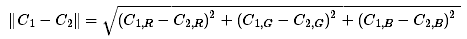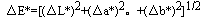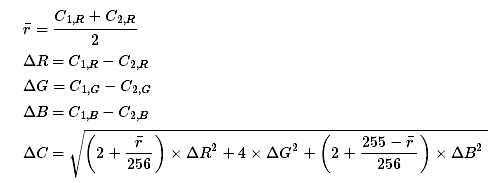# 【图像处理】颜色距离

## 1、颜色距离## 2、LAB颜色空间及色差计算

LAB颜色空间是基于人眼对颜色的感知，可以表示人眼所能感受到的所有颜色。L表示明度，A表示红绿色差，B表示蓝黄色差。两个颜色之见的色差：ΔE 表示色差，ΔL/Δa/Δb分别表示两个颜色之间在不同分量的差值。

## 3、改进的加权欧式距离typedef struct {
unsigned char r, g, b;
} RGB;

double ColourDistance(RGB e1, RGB e2)
{
long rmean = ( (long)e1.r + (long)e2.r ) / 2;
long r = (long)e1.r - (long)e2.r;
long g = (long)e1.g - (long)e2.g;
long b = (long)e1.b - (long)e2.b;
return sqrt((((512+rmean)*r*r)>>8) + 4*g*g + (((767-rmean)*b*b)>>8));
}